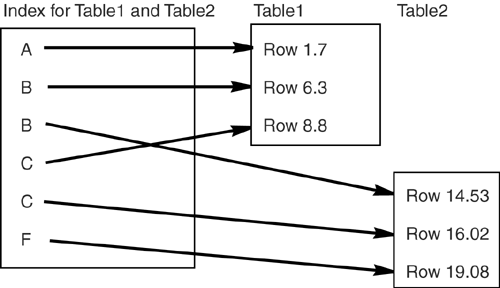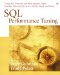# Avoid the Join Strategies

You can sometimes avoid a join by transforming an SQL statement to a simpler equivalent. For example, here is an SQL statement that could benefit from constant propagation:

` SELECT * FROM Table1, Table2    WHERE Table1.column1 = Table2.column1      AND Table1.column1 = 55 `

When the equals operator is in the restrictive clause and the same column is in both the joining clause and the restrictive clause, such a statement boils down to a non-join, like this:

` SELECT * FROM Table1, Table2    WHERE Table1.column1 = 55      AND Table2.column1 = 55 GAIN: 5/8 if both columns indexed `

There isn't a transform for every case though. The most difficult and most effective joining strategy is to do the joining in advance and store one piece of information; namely, which rows in Table1 would relate to rows in Table2 if there was an equijoin . There are several ways to blaze a trail that the DBMS can follow in such cases. We'll mention two: join indexes and composite tables.

#### Join Indexes

Some DBMSs (e.g., Informix, Microsoft, and Oracle) allow you to avoid joins by providing support for indexes that span multiple tables. Let's compare how two regular (one table per index) indexes stack up against a join index (two tables per index). Figure 5-1 shows two regular indexes, one for Table1 , the other for Table2 . In contrast, Figure 5-2 shows a join index on the same two tables.

##### Figure 5-1. Regular indexes for Table1 and Table2##### Figure 5-2. Join index for Table1 and Table2Now, suppose we do the old standby join on Table1 and Table2 :

` SELECT * FROM Table1, Table2    WHERE Table1.column1 = Table2.column1 `

When a join index is on the two tables, it's a trivial job for the DBMS to execute this statement. It needs only to scan the join index, and for each key value that points to a Table1 row, check whether the very next key has the same value and points to a Table2 row. If so, pass. In effect, this is a sort -merge join plan for which the sorting and merging have already been done! The number of I/Os needed is equal to the number of pages in the index, divided by the number of pages that the DBMS can read all at once given that this is a sequential scan.

Join indexes can be very helpful, but there is always a catch when you mingle information from two tables in one file: If you want only the information in Table1 , then access will be slower because all this irrelevant data from Table2 is in your index. On average, scan time doubles and update time more than doubles. So the rule of thumb about join indexes is:

If more than half of your queries on two tables are joins, and the join columns never change, and neither table is clustered, create a join index for the tables.

#### Composite Tables

You can also avoid joins by using a composite table . This is straightforward provided you avoid thinking of "composite indexes," which get confused with "compound indexes," which have nothing to do with "composite tables"the composite we're talking about here is a table that contains column values derived from two (or more) other tables. The composite table can thus contain join information.

Let's put this example into SQL. Assume you have three tables and one index, defined as follows :

` CREATE TABLE Table1 (    column1 INTEGER PRIMARY KEY,    column2 CHARACTER(50), ...) CREATE TABLE Table2 (    column3 INTEGER PRIMARY KEY,    column4 CHARACTER(50), ...) CREATE TABLE Composite (    column1 INTEGER,    column3 INTEGER,    column2 CHARACTER(50),    column4 CHARACTER(50), ...) CREATE UNIQUE INDEX Index1    ON Composite       (column1, column3, column2, column4) `

To keep the data in sync, if any rows of Table1 or Table2 are added or changed, the same changes must be copied to Composite . This is easy to do if your DBMS supports triggers. Then, when you need joined information from Table1 and Table2 , instead of executing this SQL statement:

` SELECT * FROM Table1, Table2    WHERE Table1.column1 = Table2.column3 `

you can do this one instead:

` SELECT * FROM Composite    WHERE column1 = column3 GAIN: 8/8 `

Doing so lets you avoid the join at this time. In effect, it's still a join, but a join that was done earlier and so doesn't cost you the same amount of time. Index Index1 is a covering index, so all the information will actually come from the compound index. But even if your DBMS doesn't provide direct support for compound indexes, you can still use the composite table.

The composite table is just a baby step toward denormalizing , so a few words on that subject are in order. (To denormalize a table means to break the normalization rules deliberately, usually in an attempt to gain speed or save space.) It's sometimes suggested that a table would benefit from denormalization "if every query on it is a join query." But as we've seen, there are many kinds of joins, and some of them have almost no effect on query speed. The usual observation in OLTP shops is that the extra INSERT/UPDATE time needed to support the composite table would be as great as the time saved when queryingso it's no savings at all unless you do a lot of queries but rarely change your data. And those are just the performance issuesthe design effects of abandoning normalization have to be considered as well. We won't discuss them heresuch issues have been well covered in most basic DBMS design texts . But if you do decide to denormalize, here are some tips:

• Copy the columns from the primary key to the foreign key, not vice versa. That way you're only violating second normal form instead of first normal form. (For more on the normal forms, see Chapter 8, "Tables.")

• Take baby steps, moving only a few columns at a time, and also leaving the same values in the original positions for a while.

• Some common advice on denormalization: OLTP shops don't need to, Decision Support System (DSS) shops do need to.

#### The Bottom Line: Avoiding Joins

If more than half of your queries on two tables are joins, and the join columns never change, and neither table is clustered, create a join index for the tables if you can.

Denormalize and use composite tables to avoid joins if you work in a DSS shop.

Don't use composite tables to avoid joins if you work in an OLTP shop.SQL Performance Tuning
ISBN: 0201791692
EAN: 2147483647
Year: 2005
Pages: 125

Similar book on Amazon